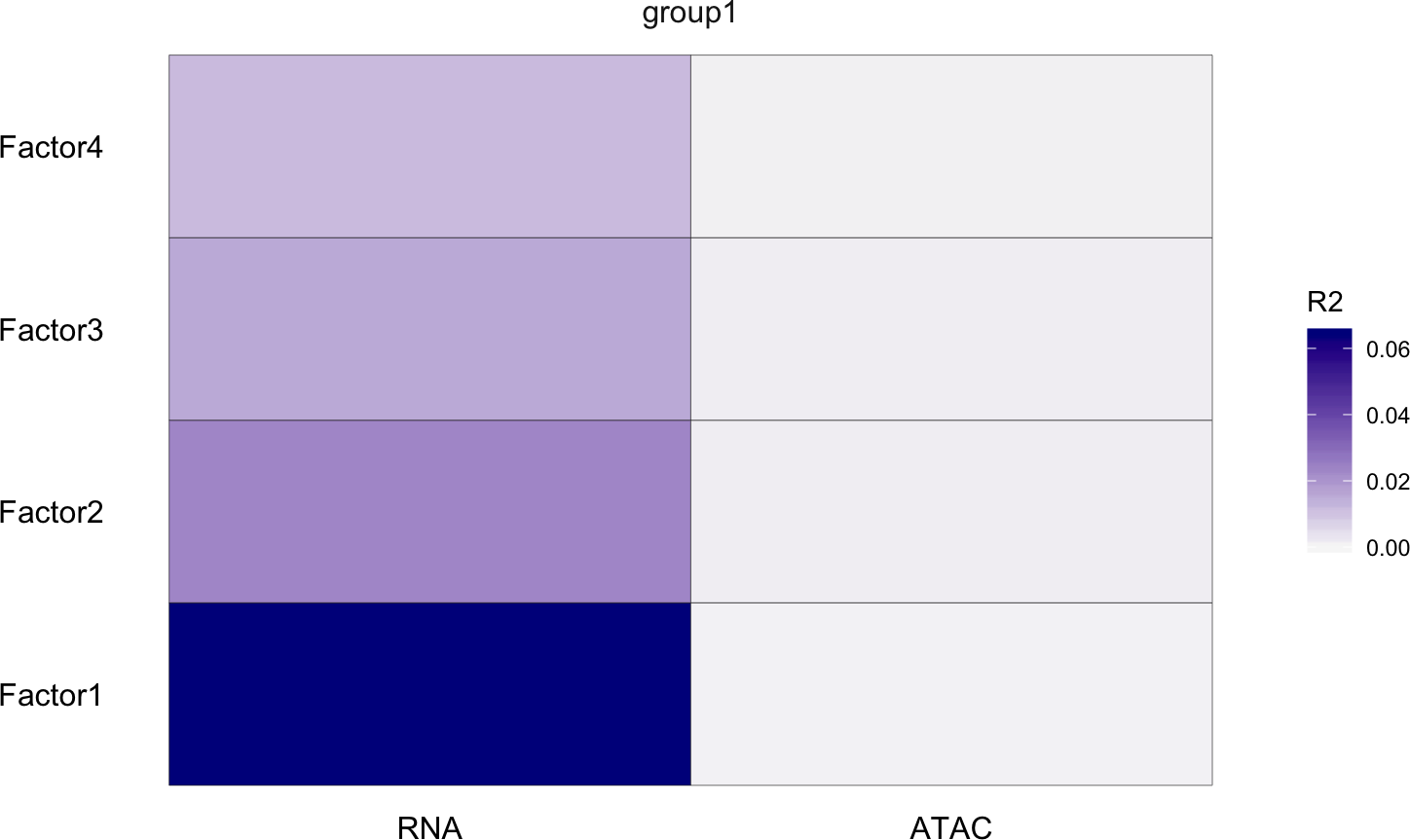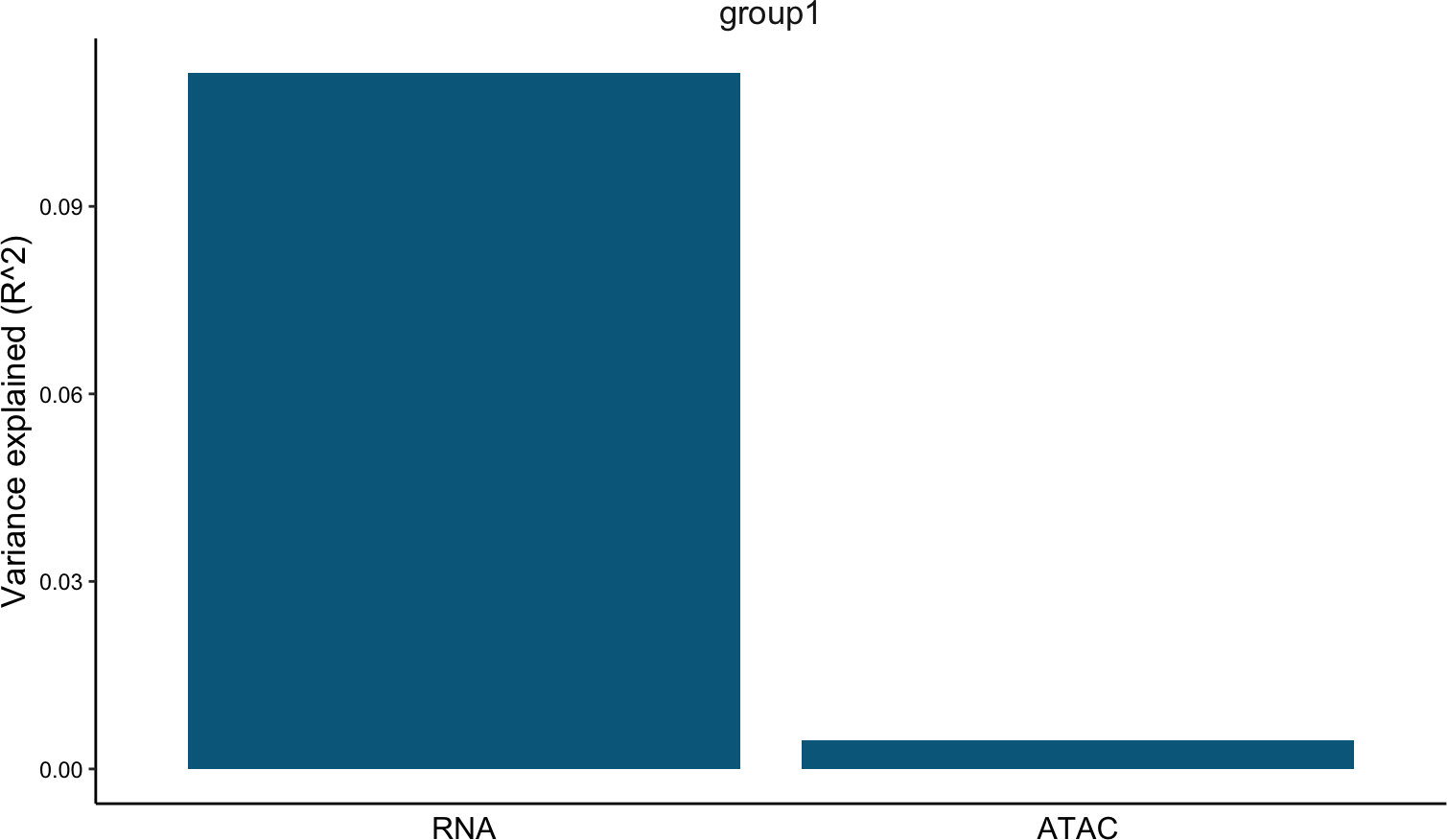# 1 Description

This vignette demonstrates how MOFA+ can be used to integrate scRNA-seq and scATAC-seq data from the same cell.
There are very few protocols to date that enable this, one of them is SNARE-seq. As a demonstration we will use a simple data set of ~1000 cells where they mixed four cell lines together: BJ, GM12878, H1 and K562.
In this setting, MOFA+ should be able to detect the (coordinated) variability in the RNA expression and in chromatin accessibiliy that determines the four different cell types.

Make sure that `MOFA2` is imported last, to avoid collisions with functions from other packages

``````library(data.table)
library(purrr)
library(Seurat)
library(ggplot2)
library(gridExtra)
library(MOFA2)``````

## 2.1 (Optional) Set up reticulate connection

``# reticulate::use_python("/Users/ricard/anaconda3/envs/base_new/bin/python", required = T)``

Load RNA modality as Seurat object

``````seurat_rna <- readRDS(url("ftp://ftp.ebi.ac.uk/pub/databases/mofa/snare_seq/seurat_rna.rds"))
dim(seurat_rna)``````
``##  5262 1047``

Load ATAC modality as Seurat object

``````# seurat_atac <- readRDS("/Users/ricard/data/Chen2019/CellLineMixture/atac/seurat.rds")
dim(seurat_atac)``````
``##  58099  1047``

``````# metadata <- fread("/Users/ricard/data/Chen2019/CellLineMixture/cell_metadata.txt")

Let’s make sure that we subset cells that match in both assays:

``````barcodes <- intersect(colnames(seurat_atac), colnames(seurat_rna))
seurat_atac <- seurat_atac[,barcodes]
seurat_rna <- seurat_rna[,barcodes]``````

## 3.1 Feature selection

Subset top 2500 most variable genes and top 10000 most variable ATAC peaks

``````# RNA
seurat_rna <- FindVariableFeatures(seurat_rna,
selection.method = "vst",
nfeatures = 2500
)
rna.features <- seurat_rna@assays\$RNA@var.features

# ATAC
seurat_atac <- FindVariableFeatures(seurat_atac,
selection.method = "disp",
nfeatures = 5000
)
atac.features <- seurat_atac@assays\$peaks@var.features``````

# 4 Train MOFA with ATAC-seq peaks

## 4.1 Create MOFA object

``````mofa <- create_mofa(list(
"RNA" = as.matrix( seurat_rna@assays\$RNA@data[rna.features,] ),
"ATAC" = as.matrix( seurat_atac@assays\$peaks@data[atac.features,] )
))

mofa``````
``````## Untrained MOFA model with the following characteristics:
##  Number of views: 2
##  Views names: RNA ATAC
##  Number of features (per view): 2500 5000
##  Number of groups: 1
##  Groups names: group1
##  Number of samples (per group): 1047``````

## 4.2 Define MOFA options

``````# Model options: let's use only 4 factors, should be enough to distinguish the four cell lines.
model_opts <- get_default_model_options(mofa)
model_opts\$num_factors <- 4

# Training options: let's use default options
train_opts <- get_default_training_options(mofa)
train_opts\$seed <- 42``````

## 4.3 Prepare the MOFA object

``````mofa <- prepare_mofa(
object = mofa,
model_options = model_opts,
training_options = train_opts
)``````

## 4.4 Run MOFA

``mofa <- run_mofa(mofa)``

## 4.5 Downstream analysis

### 4.5.2 Variance decomposition

Plot variance explained by factor

``plot_variance_explained(mofa)``Plot total variance explained per view

``plot_variance_explained(mofa, plot_total = TRUE)[]``In general, we observe that most of the variation the model captures is driven by the RNA expression and little variance is explained by the ATAC data. Why? We will explore below. First let’s have a look whether the factors make sense.

### 4.5.3 Plot factors

Looks like indeed the MOFA factors are capturing cell line variation

``plot_factors(mofa, factors=1:4, color_by = "cell_line")``